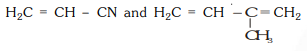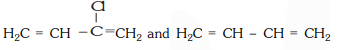Deepak Scored 45->99%ile with Bounce Back Crack Course. You can do it too!

# Buna–N synthetic rubber is a copolymer of :-

Question:

Buna–N synthetic rubber is a copolymer of :-

1. $\mathrm{H}_{2} \mathrm{C}=\mathrm{CH}-\mathrm{CN}$ and $\mathrm{H}_{2} \mathrm{C}=\mathrm{CH}-\mathrm{CH}=\mathrm{CH}_{2}$

2.3.4. $\mathrm{H}_{2} \mathrm{C}=\mathrm{CH}-\mathrm{CH}=\mathrm{CH}_{2}$ and $\mathrm{H}_{5} \mathrm{C}_{6}-\mathrm{CH}=\mathrm{CH}_{2}$

Correct Option: 1

Solution:

Buna- $\mathrm{N}$ is actually abbrebiated form, where Bu represents 1,3 -butadiene, Na represents sodium and $\mathrm{N}$ represents nitrile (acrylonitrile).

Thus, Buna-N is a copolymer of 1,3 -butadiene and acrylonitrile usually copolymer of 1,3 -butadiene, and acrylonitrile usually ploymerise in the presence of sodium.